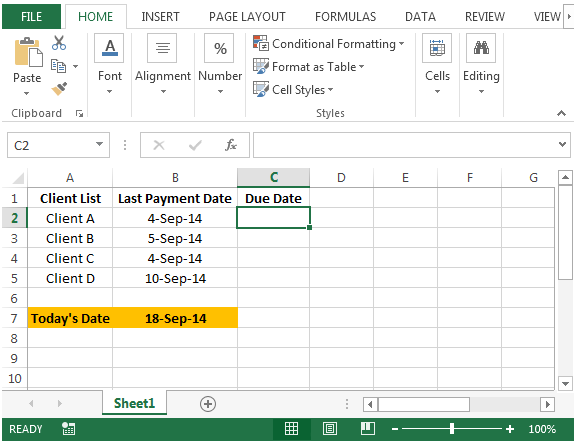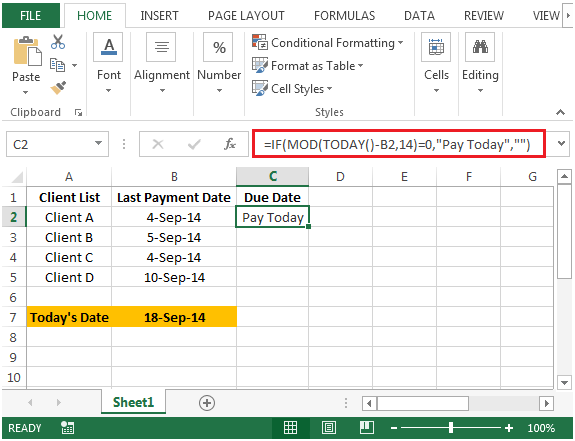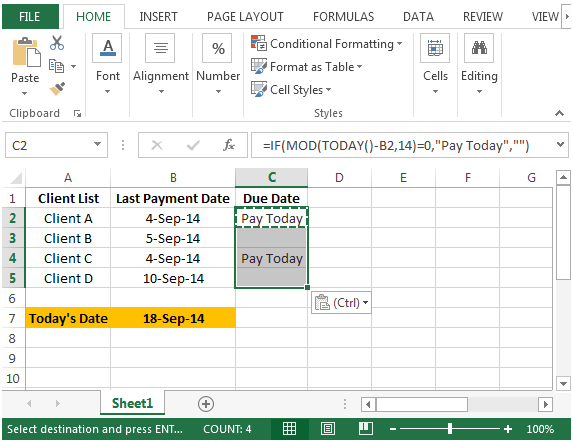# Indicating Due Payments, Based on the Current Date in Microsoft Excel 2010

In this article we will learn how to find a formula that will indicate the due payments based on the current date, we can use the combination of "IF", "MOD" & "TODAY" functions to retrieve the output in Microsoft Excel 2010.

IF function checks if the condition you specify is

TRUE or FALSE. If the condition returns TRUE then it returns preset value, and if the condition returns FALSE then it returns another preset value.

Syntax = IF(logical_test,value_if_true,value_if_false)

logical_test: Logical test will test the condition or criteria.If condition meets then it returns the preset value, and if condition does not meet then it returnsanother preset value.

value_if_true: The value that you want to be returned if this argument returns TRUE.

value_if_false: The value that you want to be returned if this argument returns FALSE
MOD: Returns the remainder after a number is divided by a divisor.

Syntax =MOD(number,divisor)

number: It is a numeric value whose remainder you want to find.

divisor: It is the number which is used to divide the number argument.
TODAY: Returns the current date formatted as a date

Syntax: =TODAY()
Let us take an example:

We have a list of Clients in column A. In column B, last payment date from every client.We need a formula that will let us know if today is the due date of the client. Calculation is based on the payments are to be collected from each client every two weeks.• In cell C2, the formula would be
• =IF(MOD(TODAY()-B2,14)=0,"Pay Today","")
• Press Enter on your keyboard.• Copying the formula from cell C2 to range C3:C5, we will get the desired result.• Client A & C have last paid on 4-Sep which is 14 days back. Hence, we have derived a formula that will indicate the due date of payment based on the current date.

## Users are saying about us...

1.CA Vikram Shankar Mathur

Nice tip.

Terms and Conditions of use

The applications/code on this site are distributed as is and without warranties or liability. In no event shall the owner of the copyrights, or the authors of the applications/code be liable for any loss of profit, any problems or any damage resulting from the use or evaluation of the applications/code.